Get Started »

# Sequences, Sums & Series

In the Wolfram Language, integer sequences are represented by lists.

Use Table to define a simple sequence:

 In:=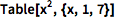⨯ `Table[x^2, {x, 1, 7}]`
 Out=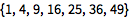Some well-known sequences are built in:

 In:=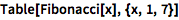⨯ `Table[Fibonacci[x], {x, 1, 7}]`
 Out=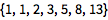Define a recursive sequence using RecurrenceTable:

(Note the use of {x,min,max} notation.)
 In:=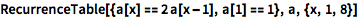⨯ `RecurrenceTable[{a[x] == 2 a[x - 1], a == 1}, a, {x, 1, 8}]`
 Out=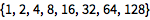Compute the Total of the sequence:

 In:=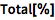⨯ `Total[%]`
 Out=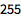Compute the Sum of a sequence from its generating function:

 In:=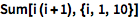⨯ `Sum[i (i + 1), {i, 1, 10}]`
 Out=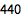Use ESCsumtESC for a fillable typeset form:

 In:=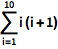⨯ ```\!\( \*UnderoverscriptBox[\(\[Sum]\), \(i = 1\), \(10\)]\(i \((i + 1)\)\)\)```
 Out=You can do indefinite and multiple sums:

 In:=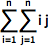⨯ ```\!\( \*UnderoverscriptBox[\(\[Sum]\), \(i = 1\), \(n\)]\( \*UnderoverscriptBox[\(\[Sum]\), \(j = 1\), \(n\)]i\ j\)\)```
 Out=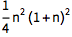Calculate a generating function for a sequence:

 In:=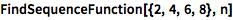⨯ `FindSequenceFunction[{2, 4, 6, 8}, n]`
 Out=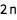Generate power series approximations to virtually any combination of built-in functions:

 In:=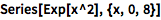⨯ `Series[Exp[x^2], {x, 0, 8}]`
 Out=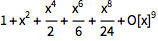O[x]9 represents higher-order terms that have been omitted; use Normal to truncate this term:

 In:=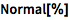⨯ `Normal[%]`
 Out=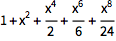Given an unknown or undefined function, Series returns a power series in terms of derivatives:

 In:=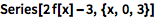⨯ `Series[2 f[x] - 3, {x, 0, 3}]`
 Out=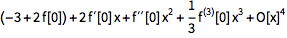Convergent series may be automatically simplified:

 In:=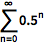⨯ ```\!\( \*UnderoverscriptBox[\(\[Sum]\), \(n = 0\), \(\[Infinity]\)] \*SuperscriptBox[\(0.5\), \(n\)]\)```
 Out=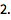QUICK REFERENCE: Integer Sequences `»`

QUICK REFERENCE: Series Expansions `»`